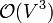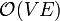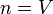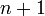# Difference between revisions of "Floyd–Warshall algorithm"

The Floyd–Warshall algorithm finds all-pairs shortest paths in a directed, weighted graph which contains no negative-weight cycles. That is, unlike Dijkstra's algorithm, it is guaranteed to correctly compute shortest paths even when some edge weights are negative. (Note however that it is still a requirement that no negative-weight cycle occurs; finding shortest paths in such a graph becomes either meaningless if non-simple paths are allowed, or computationally difficult when they are not.) With a running time of$\mathcal{O}(V^3)$, Floyd–Warshall is asymptotically optimal in dense graphs. It is outperformed by Dijkstra's algorithm or the Bellman–Ford algorithm in the single-source shortest paths problem (with running times of$\mathcal{O}(V^2)$ and$\mathcal{O}(VE)$, respectively); in the all-pairs shortest paths problem in a sparse graph, it is outperformed by repeated application of Dijkstra's algorithm and by Johnson's algorithm. Nevertheless, in small graphs (fewer than about 300 vertices), Floyd–Warshall is often the algorithm of choice, because it computes all-pairs shortest paths, handles negative weights on edges correctly while detecting negative-weight cycles, and is very easy to implement.

# The algorithm

input adj
for each k ∈ V(G)
for each i ∈ V(G)
for each j ∈ V(G)
for each k ∈ V(G)
error "Graph contains a negative-weight cycle"


At the successful conclusion of the algorithm, the adjacency matrix adj will have been transformed into a shortest-paths matrix. If the distance from each vertex to itself is initially set as infinite, this matrix will also tell us the length of the shortest path of nonzero length from each vertex back to itself.

# Theory of the algorithm

## Explanation

Floyd–Warshall is one of the most well-known examples of a dynamic programming algorithm. It consists of a single looping structure containing three nested loops and occurs in$V$ passes, where$V$ is the number of vertices in the graph. The graph should be represented as an adjacency matrix adj in order for Floyd–Warshall to be practical, and all missing edges should be assigned infinite weight. After n passes have occurred, the entry adj[u][v] should contain the length of the shortest path from u to v that uses only the first n vertices as possible intermediates along the path. At the termination of the algorithm, after all$V$ passes have occurred, the adj array ought to contain shortest paths that use any vertices whatsoever as intermediates – that which was to be determined.

## Proof of correctness

We shall prove the claim above by induction.

• At the outset, when no passes of the main outer loop have occurred, each entry adj[i][j] contains the shortest distance from i to j using only the first 0 vertices as intermediates: the weight of the edge from i to j itself.
• Now suppose$n$ passes have occurred, and that adj[i][j] contains the shortest distance from i to j using only the first$n$ vertices of the graph (By first, we mean the first to be iterated over by the outer main loop.) If$n=V$, we are done. Otherwise, the shortest path from i to j that uses only the first$n+1$ vertices of the graph either does not use the$(n+1)$th vertex, denoted k, at all, in which case its value is already known, or there exists a shorter path that does use k. To find this path, we relax the edge from i to j using k as an intermediate vertex along the path, meaning that we concatenate the i-k and k-j paths to obtain the new i-j path, and update the i-j distance by summing the i-k and k-j distances. This will always yield the shortest distance from i to j that uses k. This is because, for each of the paths i-k and k-j, if the corresponding entry in adj has not yet been updated, then it contains the shortest path for that pair that uses only vertices lower than k, which is acceptable because vertices higher than k are not being considered yet, and duplicate instances of vertex k cannot shorten the path unless negative-weight cycles are present. If it has been updated, duplicate instances of vertex k cannot lengthen the path either, since we only keep the shortest path so far found at any given time. Thus, after the$(n+1)$th pass, all pairs of shortest paths are known that do not use any vertex higher than k as an intermediate.

It is also easy to see that the loop at the end will never produce an error, because the shortest path from any vertex back to itself cannot have negative weight unless that vertex is part of a negative-weight cycle.

## Proof of detection of negative-weight cycles

If no negative-weight edges are present, which is often the case, the final loop may be omitted altogether from the algorithm, since it will never be useful. If negative-weight edges are present, then the final loop will always detect the presence of a negative-weight cycle. Suppose a negative-weight cycle exists. Then, choose any vertex k on this cycle. At the termination of the main loop, dist[k][k] will be negative, since the algorithm will inevitably have found the shortest path from k back to itself using each vertex in the graph at most once (the reason for this is explained in the previous section), which is, of course, of negative weight. (The presence of negative-weight cycles implies the presence of negative-weight simple cycles, as all non-simple cycles can be decomposed into simple cycles.) The algorithm then prints out an appropriate error message.

# Special case: Warshall's algorithm

A special case of the Floyd–Warshall algorithm is Warshall's algorithm, which tests only for reachability. If two vertices are linked by an edge, we assign the edge any finite weight (such as 0 or 1), otherwise we assign it an infinite weight. If, at the end, we find the distance from one vertex to another to be finite, then they are connected, since a path existed using only finite-weight edges (that is, ones that actually exist); otherwise, if no such path exists, the entry in the matrix will be infinity. Notice that we can replace "finite" by 1, "infinite" by 0, addition with logical AND, and min with logical OR, and preserve existing logic. This yields the following implementation of Warshall's algorithm:

input adj
for each k ∈ V(G)
for each i ∈ V(G)
for each j ∈ V(G)

(Per standard convention, and takes precedence over or.)